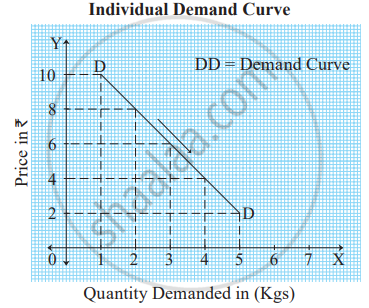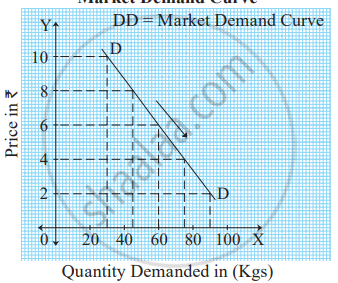# Demand Curve and Its Slope

## Demand Curve and Its Slope:

#### Individual Demand Curve :

Individual demand curve is a graphical representation of the individual demand schedule. Figure below represents an individual demand curve.In the figure above , X axis represents quantity demanded and Y axis represents the price of the commodity. The demand curve DD slopes downward from left to right, indicating an inverse relationship between price and quantity demanded.

#### Market Demand Curve :

Graphically, the market demand curve is a horizontal summation of individual demand curves. It is based on the market demand schedule.                                                          Figure below represents the market demand curve.In the figure above, X axis represents market demand and Y axis represents the price of the commodity. The market demand curve ‘DD’ slopes downward from left to right, indicating an inverse relationship between price and market demand.

Reasons justifying downward sloping demand curve are as follows :
1) Law of Diminishing Marginal Utility :

We have seen that marginal utility goes on diminishing with an increase in the stock of a commodity and vice-versa. Therefore, a consumer tends to buy more when price falls and vice-versa. This implies that demand curve is downward sloping.

#### 2) Income effect :

In the case of normal goods, when price falls, purchasing power (real income) of a consumer increases which enables him to buy more of that commodity. This is known as income effect.

3) Substitution effect:

In case of substitute goods, when the price of a commodity rises, the consumer tends to buy more of its substitute and less of that commodity
whose price has increased. This is known as substitution effect.

#### 5) New Consumers :

When the price of a commodity falls, a new consumer class appears who can now afford the commodity. Thus, total demand for commodity increases
with fall in price.

If you would like to contribute notes or other learning material, please submit them using the button below.

### Shaalaa.com

Demand and Supply [00:07:00]
S
0%